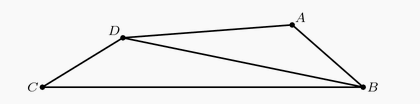###### back to index | new

Let $a$ and $b$ be positive integers satisfying $\frac{ab+1}{a+b} < \frac{3}{2}$. The maximum possible value of $\frac{a^3b^3+1}{a^3+b^3}$ is $\frac{p}{q}$, where $p$ and $q$ are relatively prime positive integers. Find $p+q$.

Let $x_1, x_2, ... , x_6$ be non-negative real numbers such that $x_1 +x_2 +x_3 +x_4 +x_5 +x_6 =1$, and $x_1 x_3 x_5 +x_2 x_4 x_6 \ge {\scriptstyle\frac{1}{540}}$. Let $p$ and $q$ be positive relatively prime integers such that $\frac{p}{q}$ is the maximum possible value of $x_1 x_2 x_3 + x_2 x_3 x_4 +x_3 x_4 x_5 +x_4 x_5 x_6 +x_5 x_6 x_1 +x_6 x_1 x_2$. Find $p+q$.

Let $(a,b,c)$ be the real solution of the system of equations $x^3 - xyz = 2$, $y^3 - xyz = 6$, $z^3 - xyz = 20$. The greatest possible value of $a^3 + b^3 + c^3$ can be written in the form $\frac {m}{n}$, where $m$ and $n$ are relatively prime positive integers. Find $m + n$.

Two of the three sides of a triangle are 20 and 15. Which of the following numbers is not a possible perimeter of the triangle?

What is the greatest possible perimeter of an isosceles triangle with sides of length $5x + 20$, $3x + 76$ and $x + 196$?

In $\triangle BAC$, $\angle BAC=40^\circ$, $AB=10$, and $AC=6$. Points $D$ and $E$ lie on $\overline{AB}$ and $\overline{AC}$ respectively. What is the minimum possible value of $BE+DE+CD$?

Real numbers $a$ and $b$ are chosen with $1 < a < b$ such that no triangle with positive area has side lengths $1$, $a$, and $b$ or $\frac{1}{b}$, $\frac{1}{a}$, and $1$. What is the smallest possible value of $b$?

$A$, $B$, $C$ are three piles of rocks. The mean weight of the rocks in $A$ is $40$ pounds, the mean weight of the rocks in $B$ is $50$ pounds, the mean weight of the rocks in the combined piles $A$ and $B$ is $43$ pounds, and the mean weight of the rocks in the combined piles $A$ and $C$ is $44$ pounds. What is the greatest possible integer value for the mean in pounds of the rocks in the combined piles $B$ and $C$?

Three distinct segments are chosen at random among the segments whose end-points are the vertices of a regular 12-gon. What is the probability that the lengths of these three segments are the three side lengths of a triangle with positive area?

Suppose that $\left|x+y\right|+\left|x-y\right|=2$. What is the maximum possible value of $x^2-6x+y^2$?

Halfway through a 100-shot archery tournament, Chelsea leads by 50 points. For each shot a bullseye scores 10 points, with other possible scores being 8, 4, 2, and 0 points. Chelsea always scores at least 4 points on each shot. If Chelsea's next $n$ shots are bullseyes she will be guaranteed victory. What is the minimum value for $n$?

Let $a$, $b$, $c$, $d$, and $e$ be positive integers with $a+b+c+d+e=2010$ and let $M$ be the largest of the sum $a+b$, $b+c$, $c+d$ and $d+e$. What is the smallest possible value of $M$?

The set of real numbers $x$ for which $\dfrac{1}{x-2009}+\dfrac{1}{x-2010}+\dfrac{1}{x-2011}\ge1$ is the union of intervals of the form $a< x \le b$. What is the sum of the lengths of these intervals?

Let $a, b$ and $c$ be numbers with $0 < a < b < c$. Which of the following is impossible?

For how many integers $x$ is the point $(x, -x)$ inside or on the circle of radius 10 centered at $(5, 5)$?

Let $a$, $b$, and $c$ be three distinct one-digit numbers. What is the maximum value of the sum of the roots of the equation $(x-a)(x-b)+(x-b)(x-c)=0$?

Nonzero real numbers $x$, $y$, $a$, and $b$ satisfy $x < a$ and $y < b$. How many of the following inequalities must be true? $\textbf{(I)}\ x+y < a+b\qquad$ $\textbf{(II)}\ x-y < a-b\qquad$ $\textbf{(III)}\ xy < ab\qquad$ $\textbf{(IV)}\ \frac{x}{y} < \frac{a}{b}$

For how many integers $x$ is the number $x^4-51x^2+50$ negative?

The length of the interval of solutions of the inequality $a \le 2x + 3 \le b$ is $10$. What is $b - a$?

A fly trapped inside a cubical box with side length $1$ meter decides to relieve its boredom by visiting each corner of the box. It will begin and end in the same corner and visit each of the other corners exactly once. To get from a corner to any other corner, it will either fly or crawl in a straight line. What is the maximum possible length, in meters, of its path?

In quadrilateral $ABCD$, $AB = 5$, $BC = 17$, $CD = 5$, $DA = 9$, and $BD$ is an integer. What is $BD$?In a triangle with integer side lengths, one side is three times as long as a second side, and the length of the third side is 15. What is the greatest possible perimeter of the triangle?

If $0 \le a_1 \le a_2 \le a_3 \le \cdots \le a_n \le 1$, find the maximum value of $$\sum_{1 \le i < j \le n}(a_j-a_i+1)^2+4 \sum_{i=i}^n a_i^2$$

What percent of the interval with endpoints \u22125 and 5 consists of real numbers $x$ satisfying the inequality $x + 1 > \frac{8}{x -1}$?

Shaina has one stick of length $a$ cm and another of length $b$ cm, where $a \ne b$. She needs a third stick with length strictly between 8 cm and 26 cm to make the third side of a triangle. What is the product $ab$?